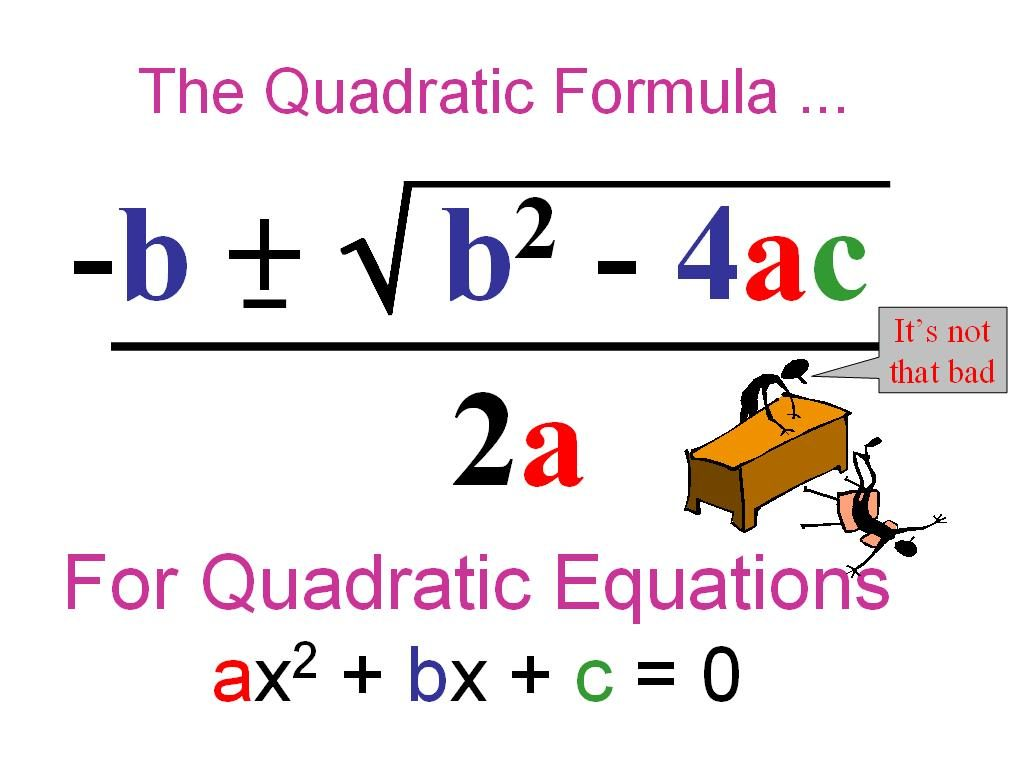# SOLVING QUADRATIC EQUATIONS USING QUADRATIC FORMULA METHOD (VIDEO)Watch the video here on how to solve quadratic equations using Quadratic formula method:

After watching the video, try and solve the following quadratic equations using Quadratic formula method:

(1) x2 + 5 + 6x  = 0                                          (2) x2 – 2x  – 3 = 0                                         (3) 2x2 – 5x  – 3 = 0                         (4) 2x2 – 3x – 20 = 0

(Photo Credit:  msmcculloughsmathsclass.blogspot.com)